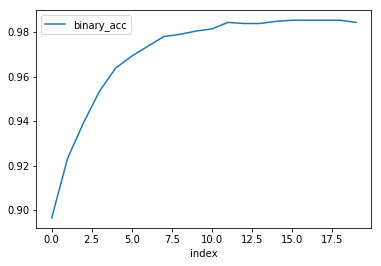# Trial History and Replay¶

This guide will give a breif overview of the history returned by a Trial from a call to fit or evaluate.

Note: The easiest way to use this tutorial is as a colab notebook, which allows you to dive in with no setup.

## Install Torchbearer¶

First we install torchbearer if needed.

In :
try:
import torchbearer
except:
!pip install -q torchbearer
import torchbearer

print(torchbearer.__version__)

0.4.0.dev


## A Simple Example¶

We first create some data and a simple model to train on.

In :
import torch
import torch.nn as nn

class BasicModel(nn.Module):
def __init__(self):
super(BasicModel, self).__init__()
self.linear1 = nn.Linear(100, 25)
self.linear2 = nn.Linear(25, 1)

def forward(self, x):
x = self.linear1(x)
x = self.linear2(x)

import numpy as np
from sklearn.datasets.samples_generator import make_blobs

X, Y = make_blobs(n_samples=2048, n_features=100, centers=2, cluster_std=10, random_state=1)
X = (X - X.mean()) / X.std()
Y[np.where(Y == 0)] = -1
X, Y = torch.FloatTensor(X), torch.FloatTensor(Y)


Next, we'll run the model for a few epochs to obtain a history.

In :
import torch.optim as optim
import torch.nn.functional as F

from torchbearer import Trial

model = BasicModel()

optimizer = optim.SGD(filter(lambda p: p.requires_grad, model.parameters()), lr=0.001)
trial = Trial(model, optimizer=optimizer, criterion=F.binary_cross_entropy,
metrics=['mse', 'acc', 'loss'])
trial.with_train_generator(traingen)
history = trial.run(epochs=20, verbose=1)


## The History¶

The history is a list of metric dictionaries from each epoch of training. History also includes the number of training and validation steps from each epoch. Let's take a look

In :
print(len(history))
print(history)

20
{'running_mse': 0.9208962321281433, 'running_binary_acc': 0.960156261920929, 'running_loss': -0.019676249474287033, 'mse': 0.8676411509513855, 'binary_acc': 0.96923828125, 'loss': -0.15272381901741028, 'train_steps': 16, 'validation_steps': None}


### With Pandas¶

Suppose that we wanted to use pandas to plot our training progress or similar, we could do that with the following

In :
import pandas as pd

frame = pd.DataFrame.from_records(history)
print(frame)

    binary_acc      loss       mse  running_binary_acc  running_loss  \
0     0.896484  0.316458  1.067553            0.887784      0.334222
1     0.923340  0.225443  1.025509            0.904803      0.286757
2     0.939453  0.133693  0.984494            0.916061      0.240595
3     0.953613  0.040475  0.944467            0.933594      0.167838
4     0.963867 -0.054826  0.905476            0.949063      0.074993
5     0.969238 -0.152724  0.867641            0.960156     -0.019676
6     0.973633 -0.253658  0.831138            0.967812     -0.116728
7     0.978027 -0.358019  0.796175            0.972656     -0.216635
8     0.979004 -0.466167  0.762976            0.975781     -0.319814
9     0.980469 -0.578459  0.731756            0.978750     -0.426640
10    0.981445 -0.695270  0.702708            0.980313     -0.537480
11    0.984375 -0.817010  0.675986            0.982188     -0.652704
12    0.983887 -0.944141  0.651693            0.983594     -0.772715
13    0.983887 -1.077197  0.629881            0.984375     -0.897960
14    0.984863 -1.216788  0.610547            0.984531     -1.028948
15    0.985352 -1.363614  0.593634            0.985000     -1.166263
16    0.985352 -1.518468  0.579039            0.985469     -1.310573
17    0.985352 -1.682242  0.566622            0.985625     -1.462638
18    0.985352 -1.855926  0.556210            0.985625     -1.623313
19    0.984375 -2.040609  0.547611            0.985469     -1.793551

running_mse  train_steps validation_steps
0      1.077145           16             None
1      1.054281           16             None
2      1.033050           16             None
3      1.000690           16             None
4      0.960275           16             None
5      0.920896           16             None
6      0.882645           16             None
7      0.845669           16             None
8      0.810160           16             None
9      0.776331           16             None
10     0.744397           16             None
11     0.714554           16             None
12     0.686969           16             None
13     0.661763           16             None
14     0.639006           16             None
15     0.618716           16             None
16     0.600858           16             None
17     0.585348           16             None
18     0.572062           16             None
19     0.560841           16             None


We can now use all of the built-in pandas functions, such as plotting

In :
%matplotlib inline
frame.reset_index().plot('index', 'binary_acc')

Out:
<matplotlib.axes._subplots.AxesSubplot at 0x7fbb6e59aa20>## Replay a Trial¶

One of the perks of history is the ability to replay a trial. We'll look at two of the replay options here. First we can just replay the whole training process, this time with a different verbosity.

In :
_ = trial.replay(verbose=2)


This may be more output than we desire, and so we can instead use the one_batch flag to just simulate one batch per epoch.

In :
_ = trial.replay(verbose=2, one_batch=True)


So that's history and replaying in torchbearer. Be sure to have a look at our other examples at pytorchbearer.org.

In [ ]: